# Anti-Swing Rejection Based on PID Controller Optimized by Firefly Algorithm

Anti-Swing Rejection Based on PID Controller Optimized by Firefly Algorithm
Electrical and Electron department, Faculty of Engineering, University of Al-Qadisiyah, Diwaniyah 00964, Iraq

Roads and Transports Department, Faculty of Engineering, University of Al-Qadisiyah, Diwaniyah 00964, Iraq

Corresponding Author Email:
amjed.saleh@qu.edu.iq
Page:
347-351
|
DOI:
https://doi.org/10.18280/mmep.100140
2 December 2022
|
Revised:
20 January 2023
|
Accepted:
25 January 2023
|
Available online:
28 February 2023
| Citation

OPEN ACCESS

Abstract:

Gantry crane systems are often used in a variety of industrial applications to move and raise enormous weights. The process of transferring loads and weights in crane systems has always occupied the interest of researchers because of its importance in maintaining the safety of workers on the one hand and preserving the load itself from damage as a result of its swing and the possibility of it colliding with surrounding objects on the other hand. This paper's primary goal is to use a PID controller to regulate the position and swing of a GCS utilizing genetic algorithms (GA) and firefly algorithms (FA). Analytical techniques are used in the mathematical model, while Simulink in MATLAB is used in the PID model. This technique gave excellent results compared to either using the genetic algorithms (GA).

Keywords:

gantry crane system (GCS), firefly algorithm (FA), PID controller, genetic algorithms (GA), Optimization

1. Introduction

The crane system is an instrument or piece of equipment that allows you to precisely lift and transfer large goods from one point to another. Because of the dominating benefits of high payload capacity, outstanding flexibility, and time–saving capabilities, a gantry crane system (GCS) is regarded as one of the most critical equipment in handling heavy load items in industries. As for the crane's acceleration and deceleration during movement, the loads swing back and forth. As a result, when the GCS is propelled into motion, it is susceptible to disturbances such as wind, waves, and environmental distortion . Excessive load swing owing to crane motion and difficulties in trolley placement on the correct trajectory with a rapid response time are two of these challenges .

Several gantry crane position and anti-swing control algorithms have been developed and implemented in several published publications. For industrial three-dimensional overhead cranes, [3, 4] suggested a novel fuzzy logic anti-swing control. The proposed control ensures both precise position control and quick load swing damping for the crane's simultaneous travel, traverse, and hoisting actions, according to the experimental findings. To improve the PID parameters in the gantry crane system, meta-heuristic approaches are used. To regulate the position and sway of an overhead crane, the LQR controller's settings are optimized using a genetic algorithm . A combination of PID and fuzzy control creates a stable overhead crane controller . To lower the payload swing angle, a PID+Q controller was created . Abdullah et al.  propose a Hybrid Control Scheme (HCS) based on energy balance and fuzzy logic controllers to achieve RIP swing up and stabilization control. The extended method RRT, which is asymptotically optimum, is presented by Karaman and Frazzoli . The paper  presents Overhead cranes were subjected to an adaptive-fuzzy SMC for the robust anti-sway pursuit under both system uncertainty and actuator nonlinearity. The FLC parameters are automatically tuned using meta-heuristic optimization techniques. To increase the efficacy of FLC design, the scaling factors of FLC are optimized using three well-known meta-heuristic techniques . The paper  is used to regulate the motion of the location of the overhead crane utilizing a PID controller and optimization methods such as Genetic Algorithms (GA) and Bee Algorithms (BA).

In this work, the proportional integral derivative (PID) controller parameters for the Nonlinear Gantry Crane System are modified using the firefly algorithm (FA) and genetic algorithm (GA). It has been demonstrated in tests that FA is more potent and performs better than the Genetic Algorithm (GA). To discover the PID parameters, it has a flexible and adaptable property. By decreasing or maximizing the factors involved in the issues, optimization is a method of discovering the optimal solution to make something as functional and effective as feasible.

2. Gantry Crane Dynamic Model

Figure 1 shows a gantry crane system, it consists of a load, a bridge, a trolley, electric motors, a cargo rope, and a gear motor [13-15]. The most practical tool for calculating the gantry crane model is Lagrange's equation . The trolley displacement from a reference location x(ref), the payload swing angle θ, and the steel wire elongation $l$ are the three key factors that affect the gantry crane system. The following is a description of the system's dynamics .

$\frac{\mathrm{d}}{\mathrm{dt}}\left[\frac{\partial \mathrm{L}}{\partial \dot{\mathrm{q}}_1}\right]-\frac{\partial \mathrm{L}}{\partial \mathrm{q}_{\mathrm{i}}}=\mathrm{Q}_{\mathrm{i}}$     (1)

Lagrangian function, non - conservative conceptual powers, and independence generalized coordinates are represented by L, Qi, and qi, respectively. It can be expressed in writing in a variety of ways.

$L=T-P$     (2)

The kinetic and potential energies, respectively, are T and P. This relationship is used to calculate how flexible coordinates are connected. The following are the formulas for kinetic, potential energies.

$\begin{gathered}L=\frac{1}{2}\left(m_1 \dot{x^2}+m_2 \dot{x}^2+m_1 l^2 \dot{\theta}^2\right)+m_1 \dot{x} \dot{\theta} l \cos \theta \\ \left.+m_1 g l \cos \theta\right)\end{gathered}$     (3)

Because a dynamic DC motor is incorporated in this gantry crane model, differential equations must be used and their implications are generated. Taking the dynamic DC motor into account, a thorough nonlinear differential equation of the GCS can be acquired as follows .

$\begin{gathered}V=\left[\frac{R B r_p}{K_T z}+\frac{K_E z}{r_p}\right]+\left[\frac{R B r_p}{K_T z}\right]\left(m_1 l\right)\left[\ddot{\theta} \cos \theta-\dot{\theta}^2 \sin \theta\right]+ \\ {\left[\frac{R B r_p}{K_T z}\right]\left(m_1+m_2\right) \ddot{x}}\end{gathered}$     (4)

$m_1 l^2 \ddot{\theta}+m_1 l \ddot{x} \cos \theta+m_1 g l \sin \theta=0$     (5)

## 40-figure1.jpg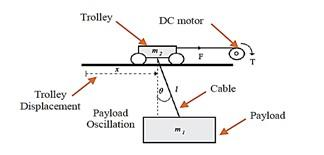Figure 1. Gantry crane system 

3. Firefly Algorithm

This relatively new algorithm has succeeded in refining and improving a variety of optimization issues, including image processing , cutting tool selection , motor control , energy management for electric cars , robot control , and steel building design . Yang devised the Firefly algorithm, which Krishnanad initially suggested in 2005 , To begin with, fireflies are all unisex. As a result, regardless of gender, any firefly can be attracted to other fireflies. Second, attraction is proportional to intensity, which is a function of the distance between the firefly in question and the others. Finally, the value of the cost function of the problem stated determines the luminance or luminous intensity of a firefly. The FA method may be expressed mathematically using the equations below .

$I(r)=I_0 e^{-\gamma r^2}$     (6)

where, (I0) denotes the source point's intensity and light absorption coefficient t)γ). In the same way, the brightness (β), may be calculated using Eq. (7).

$\beta=\beta_0 e^{-\gamma r^2}$     (7)

The intensity of light is inversely proportional to the square of the distance, say (r), from the source, according to simple physics. Additionally, when light passes through a substance with a light absorption coefficient γ.

Eq. (8) gives a generalized brightness function for (ω≥1). In fact, you may use any monotonically declining function .

$\beta=\beta_0 e^{-\gamma \omega}$     (8)

The technique assigns a light intensity to a randomly generated viable s as a result, Using the update process in Eq. (9), if firefly (j) is more shining than firefly (i), firefly (i) will approach firefly (j).

$x_i=x_i+\beta_0 e^{-\gamma r^2} i j\left(x_j-x_i\right)+\alpha(\varepsilon(\enspace)-0.5)$     (9)

where, β0 is xj's allure when r equals 0,  recommends β0=1, is an algorithm parameter for the step length of the random movement, is an algorithm parameter for the degree to which the updating process depends on the separation between the two fireflies, and (ε ( ) ) is a uniformly distributed random vector with values ranging from 0 to 1. The second formula in Eq. (10) will be deleted to find the shiniest firefly, xb, as shown in Eq. (10) .

$x_b=x_b+\alpha(\varepsilon(\enspace)-0.5)$     (10)

4. Simulation Results and Discussions

Anti-swing control's major goal is to transfer the load as quickly as possible without creating an excessive amount of swing in the end position. Figure 2 shows a simplified block schematic of the control strategy used in the research. The PID controller's control objectives include reducing payload oscillation and positioning control of trolley displacement . PID controllers have a straightforward structure that anyone with a foundational understanding of control engineering can easily comprehend and modify . Measure of the system error is used as a performance criterion in order to reduce the system error by predicting the inputs . A collection of excellent control settings for performance criterion minimization can result in a satisfactory step response in the time domain. Eq. (11) Refers to Integral of Time-weighted Absolute Error (ITAE). The (ITAE) is frequently mentioned as a useful tuning criterion for obtaining controller PID settings.

$\operatorname{ITAE}=\int_0^{\infty} t|e(t)| d t$     (11)

## 40-figure2.png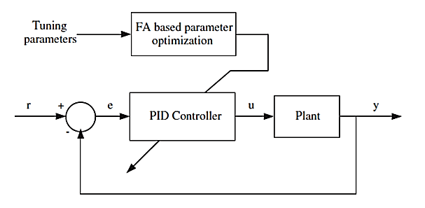Figure 2. The parameter scheduling mechanism used by PID controllers

Simulink is used to build the gantry crane system's nonlinear model, which includes trolley and sway PID controllers based on FA. The suggested control scheme's goal is to manage the trolley position (Xact) such that it advances to the reference position (Xref) as rapidly as possible without causing the load (act) to sway excessively.

Figure 3 shows the designed control scheme, which consists of a trolley controller and a sway controller. Table 1 shows the GCS system's parameter values, which are based on the study .

## 40-figure3.png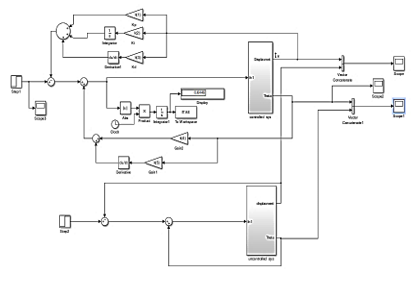Figure 3. Nonlinear GCS Simulink block model

Table 1. Parameters of the system 

 Parameters Value(unit) Parameters Value(unit) Payload (m1) 0.5 kg Resistance (R) 2.6$(\Omega)$ Trolley mass (m2) 2.0 kg Torque constant (KT) 0.007 Nm/A Cable length (l) 0.5 m Electric constant KE 0.007 Vs/rad Gravitational (g) 9.81 m/s2 Radius of pulley (rp) 0.02 m Damping coefficient (B) 0.001 Ns/m Gear ration (z) 0.15

The GCS simulations were carried out in MATLAB/Simulink. Figures 4, 5 display the simulation results for the trolley displacement and swing angle based on FA. The Firefly Algorithm parameters are configured with the best values of β0=0.2, α=0.2, γ=1, the number of fireflies is 25, and the number of iterations is 150.

## 40-figure4.png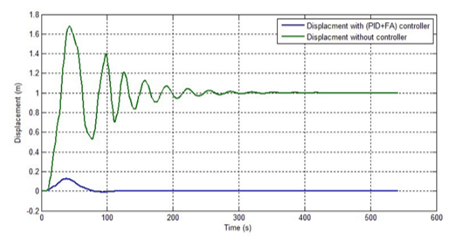Figure 4. Trolley displacement response based on FA+PID

Figures 6 and 7 display the simulation results for the trolley displacement and swing angle based on GA. The FA and GA were given the same amount of runs. When compared to the GA controller, the FA greatly reduced oscillation that occurred during the trolley motion. The FA reduced the maximum peak overshoot and the steady-state error that happened during the simulation is finally eliminated through FA. According to the findings, the FA has a quicker velocity response and a shorter settling time.

## 40-figure5.png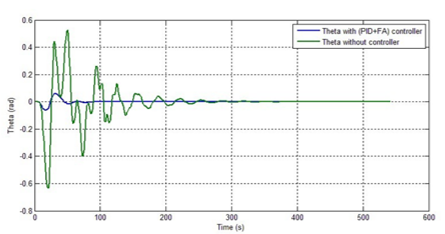Figure 5. Theta response based on FA+PID

## 40-figure6.png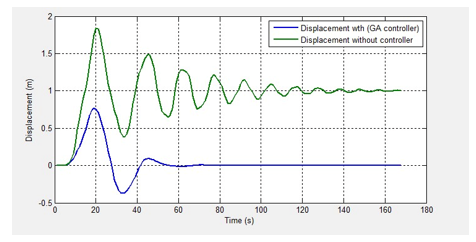Figure 6. Trolley displacement response based on GA+PID

## 40-figure7.pngFigure 7. Payload oscillation response based on GA+PID

Table 2. Displacement response data

 Performance data Based FA Based GA Rise time 1.1407e-5 5.1234e-5 Overshoot 3.364e6 2.07e7 Settling time 6.6642 15.6599

Table 3. Theta response data

 Performance data Based FA Based GA Rise time 6.7803e-6 9.166e-5 Overshoot 1.092e6 7.118e6 Settling time 5.0048 16.3394

Tables 2 and 3 indicate t the system specifications that were derived using the PID controller based FA and GA algorithm are compiled. Trolley displacement performance is shown in terms of rising time, overshoot, and settling time, whereas payload oscillation is shown as the duration of one cycle oscillation and maximum angle of oscillation. The controller's numerical parameters for displacement and theta response.

5. Conclusions

In this research paper, the main goal is achieved by reducing the angle swing and load movement time of GCS by adjusting the PID controller parameters using the firefly algorithm (FA) and comparing its result with (GA) as one of the successful and well-known techniques. One of the most important challenges that were encountered is the time it takes to implement the number of iterations on the computer, so future research will be directed towards the use of hybrid algorithms such as hybrid firefly algorithms (HFA), firefly plus cross-entropy method (FA+CE) and more other techniques that add more features to the original techniques, reduce the time spent for iterations and improve performance in general and examined it on anther and more complicated systems.

Acknowledgment

We would like to offer our heartfelt gratitude to those writers whose articles in the field of FA have been published in a variety of publications and conference proceedings.

References

 Jaafar, H.I., Mohamed, Z., Abidin, A.F.Z., Ab Ghani, Z. (2012). PSO-tuned PID controller for a nonlinear gantry crane system. In 2012 IEEE International Conference on Control System, Computing and Engineering, Penang, Malaysia, pp. 515-519.‏ https://doi.org/10.1109/ICCSCE.2012.6487200

 Ramli, L., Mohamed, Z., Abdullahi, A.M., Jaafar, H.I., Lazim, I.M. (2017). Control strategies for crane systems: A comprehensive review. Mechanical Systems and Signal Processing, 95: 1-23.‏ https://doi.org/10.1016/j.ymssp.2017.03.015

 Lee, H.H., Cho, S.K. (2001). A new fuzzy-logic anti-swing control for industrial three-dimensional overhead cranes. Proceedings 2001 ICRA. IEEE International Conference on Robotics and Automation (Cat. No.01CH37164), Korea (South).‏ https://doi.org/10.1109/ROBOT.2001.933070

 Chang, C.Y., Chiang, K.H., Hsu, S.W. (2005). Fuzzy controller for the 3-D overhead crane system. In 2005 IEEE International Conference on Robotics and Biomimetics-ROBIO, pp. 724-729. https://doi.org/10.1109/ROBIO.2005.246358

 Önen, Ü., Çakan, A. (2017). Anti-swing control of an overhead crane by using genetic algorithm based LQR. International Journal of Engineering and Computer Science, 6(6).‏ https://doi.org/10.18535/ijecs/v6i6.12

 Zhou, Q., Wang, K., Xiong, X., Zhao, J. (2021). Optimization of bridge crane control system using fuzzy PID control and speed control of frequency converter. Journal of Physics: Conference Series, 1802(3): 032007. https://doi.org/10.1088/1742-6596/1802/3/032007

 Matsuo, T., Yoshino, R., Suemitsu, H., Nakano, K. (2004). Nominal performance recovery by PID+ Q controller and its application to antisway control of crane lifter with visual feedback. IEEE Transactions on Control Systems Technology, 12(1): 156-166.‏ https://doi.org/10.1109/TCST.2003.821964

 Abdullah, M., Amin, A.A., Iqbal, S., Mahmood-ul-Hasan, K. (2021). Swing up and stabilization control of rotary inverted pendulum based on energy balance, fuzzy logic, and LQR controllers. Measurement and Control, 54(9-10): 1356-1370.‏ https://doi.org/10.1177/00202940211035406

 Karaman, S., Frazzoli, E. (2011). Sampling-based algorithms for optimal motion planning. The International Journal of Robotics Research, 30(7): 846-894.‏ https://doi.org/10.1177/0278364911406761

 Park, M.S., Chwa, D., Hong, S.K. (2008). Antisway tracking control of overhead cranes with system uncertainty and actuator nonlinearity using an adaptive fuzzy sliding-mode control. IEEE Transactions on Industrial Electronics, 55(11): 3972-3984.‏ https://doi.org/10.1109/TIE.2008.2004385

 Solihin, M.I., Chuan, C.Y., Astuti, W. (2020). Optimization of fuzzy logic controller parameters using modern meta-heuristic algorithm for gantry crane system (GCS). Materials Today: Proceedings, 29: 168-172.‏ https://doi.org/10.1016/j.matpr.2020.05.641

 Esleman, E.A., Önal, G., Kalyoncu, M. (2021). Optimal PID and fuzzy logic based position controller design of an overhead crane using the Bees Algorithm. SN Applied Sciences, 3(10): 1-13.‏

 Jaafar, H.I., Latif, N.A., Kassim, A.M., Abidin, A.F.Z., Hussien, S.Y.S., Aras, M.S.M. (2015). Motion control of nonlinear gantry crane system via priority-based fitness scheme in firefly algorithm. In AIP Conference Proceedings, 1660(1): 070031.‏ https://doi.org/10.1063/1.4915749

 Suvorov, V.A., Bahrami, M.R., Akchurin, E.E., Chukalkin, I.A., Ermakov, S.A., Kan, S.A. (2021). Anti sway tuned control of gantry cranes. SN Applied Sciences, 3(8): 1-10.‏ https://doi.org/10.1007/s42452-021-04719-w

 Solihin, M.I., Kamal, M.A.S., Legowo, A. (2008). Optimal PID controller tuning of automatic gantry crane using PSO algorithm. In 2008 5th International Symposium on Mechatronics and Its Applications, Amman, Jordan, pp. 1-5. https://doi.org/10.1109/ISMA.2008.4648804

 Ranjbari, L., Shirdel, A.H., Aslahi-Shahri, M., Anbari, S., Ebrahimi, A., Darvishi, M., Alizadeh, M., Rahmani, R., Seyedmahmoudian, M. (2015). Designing precision fuzzy controller for load swing of an overhead crane. Neural Computing and Applications, 26(7): 1555-1560.‏ https://doi.org/10.1007/s00521-015-1825-z

 Jaafar, H.I., Mohamed, Z., Jamian, J.J., Abidin, A.F.Z., Kassim, A.M., Ab Ghani, Z. (2013). Dynamic behaviour of a nonlinear gantry crane system. Procedia Technology, 11: 419-425.‏ https://doi.org/10.1016/j.protcy.2013.12.211

 Tuba, M., Bacanin, N. (2014). JPEG quantization tables selection by the firefly algorithm. In 2014 International Conference on Multimedia Computing and Systems (ICMCS), Marrakech, Morocco, pp. 153-158. https://doi.org/10.1109/ICMCS.2014.6911315

 Zubair, A.F., Mansor, M.S.A. (2019). Embedding firefly algorithm in optimization of CAPP turning machining parameters for cutting tool selections. Computers & Industrial Engineering, 135: 317-325. https://doi.org/10.1016/j.cie.2019.06.006

 Anshory, I., Hadidjaja, D., Jakaria, R.B. (2020). Bldc motor: Modeling and optimization speed control using firefly algorithm. Dinamik, 25(2): 51-58.

 Kayalvizhi, E., Karthikeyan, A., Arunarasi, J. (2015). An optimal energy management system for electric vehicles using firefly optimization algorithm based dynamic EDF scheduling. International Journal of Engineering and Technology, 7(4).

 Nedic, N., Stojanovic, V., Djordjevic, V. (2015). Optimal control of hydraulically driven parallel robot platform based on firefly algorithm. Nonlinear Dynamics, 82(3): 1457-1473. https://doi.org/10.1007/s11071-015-2252-5

 Carbas, S. (2020). Enhanced firefly algorithm for optimum steel construction design. In Applications of Firefly Algorithm and Its Variants, Springer, Singapore, 119-146. https://doi.org/10.1007/978-981-15-0306-1_6

 Yang, X.S. (2010). Nature-Inspired Metaheuristic Algorithms. Luniver Press.‏

 Yang, X.S. (200). Firefly Algorithms for Multimodal Optimization. In: Watanabe, O., Zeugmann, T. (eds) Stochastic Algorithms: Foundations and Applications. SAGA 2009. Lecture Notes in Computer Science, vol 5792. Springer, Berlin, Heidelberg. https://doi.org/10.1007/978-3-642-04944-6_14

 Okubanjo, A., Oyetola, O., Adekomaya, O. (2018). Vision based control of gantry crane system. Eskişehir Technical University Journal of Science and Technology A-Applied Sciences and Engineering, 19(4): 1023-1032.‏ https://doi.org/10.18038/aubtda.420980

 Deniz, F.N. (2022). An approach to preserve optimality in implementation of fractional order PID controllers considering approximation methods. In 2022 International Symposium on Multidisciplinary Studies and Innovative Technologies (ISMSIT), Ankara, Turkey, pp. 402-407. https://doi.org/10.1109/ISMSIT56059.2022.9932718

 Azmi, N.I.M., Yahya, N.M., Fu, H.J., Yusoff, W.A.W. (2019). Optimization of the PID-PD parameters of the overhead crane control system by using PSO algorithm. In MATEC Web of Conferences, 255: 04001. https://doi.org/10.1051/matecconf/201925504001